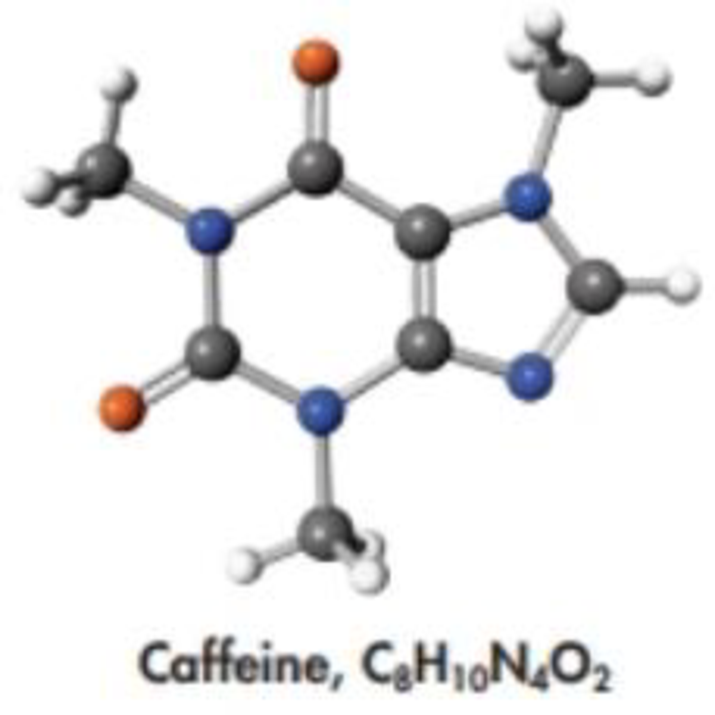Chapter 23, Problem 101GQ

Chapter
Section
Textbook Problem

Review the opening photograph about chocolate (which shows the structure of an active ingredient, theobromine) and then answer the following questions.(a) How do theobromine and caffeine differ structurally?(b) A 5.00-g sample of Hershey’s cocoa contains 2.16% theobromine. What is the mass of the compound in the sample?

(a)

Interpretation Introduction

Interpretation:

The structural different has to be explained between caffeine and theobromine.

Concept Introduction:

Theobromine also found in chocolate, it has molecular formula C7H8N4O2. The major strcutural difference between caffeine and theobromine is that one of the nitrogen atom is bonded with hydrogen atom instead of methyl group.

Explanation

The structural difference between caffeine and theobromine can be explained by drawing their structures. Both the compounds are found in chocolate as constituents. The molecular formula of caffeine is C8H10N4O2 while molecular formula of theobromine is C7H

(b)

Interpretation Introduction

Interpretation:

the mass of compound has to be calculated when 5.00 g heobromine sample of Harshey’s cocoa contains 2.16 % theobromine.

Concept Introduction:

The mass of the theobromine can be calculated by using the following expression,

mass of theobromine = (weight of sample)(percentage of theobromine)100 g           (1)

Still sussing out bartleby?

Check out a sample textbook solution.

See a sample solution

The Solution to Your Study Problems

Bartleby provides explanations to thousands of textbook problems written by our experts, many with advanced degrees!

Get Started

The smallest unit of any substance is the _____. a. atom b. molecule c. cell

Biology: The Unity and Diversity of Life (MindTap Course List)

. state the third law of thermodynamics.

Chemistry for Engineering Students

Define carbohydrate.

Introduction to General, Organic and Biochemistry

Why does a diatomic gas have a greater energy content per mole than a monatomic gas at the same temperature?

Physics for Scientists and Engineers, Technology Update (No access codes included)